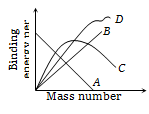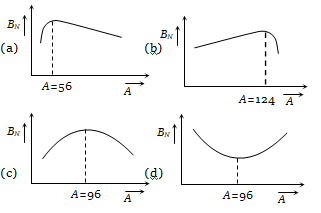Binding energy per nucleon plot against the mass number for stable nuclei is shown in the figure. Which curve is correct ?1. A
2. B
3. C
4. D

Concept Questions :-

Nuclear binding energy

Difficulty Level:

The dependence of binding energy per nucleon, ${\mathrm{B}}_{\mathrm{N}}$ on the mass number, A, is represented byConcept Questions :-

Nuclear binding energy
High Yielding Test Series + Question Bank - NEET 2020

Difficulty Level:

The ratio of radii of nuclei ${}_{13}{}^{27}\mathrm{Al}$ and ${}_{52}{}^{\mathrm{A}}\mathrm{X}$ is 3 : 5. The number of neutrons in the nuclei of X will be
(a) 52              (b) 73
(c) 125             (d) 13

Concept Questions :-

Mass-energy equivalent
High Yielding Test Series + Question Bank - NEET 2020

Difficulty Level:

A radioactive sample is $\mathrm{\alpha }$-emitter with half life 138.6 days is observed by a student to have 2000 disintegration/sec. The number of radioactive nuclei for given activity are
(a) $3.45×{10}^{10}$               (b) $1×{10}^{10}$
(c) $3.45×{10}^{15}$               (d) $2.75×{10}^{11}$

Concept Questions :-

High Yielding Test Series + Question Bank - NEET 2020

Difficulty Level:

Consider a hydrogen like atom whose energy in nth exicited state is given by  when this excited atom makes a transition from excited state to ground state, most energetic photons have energy ${\mathrm{E}}_{\mathrm{max}}$ = 52.224 eV and least energetic photons have energy ${\mathrm{E}}_{\mathrm{min}}$ = 1.224 eV. The atomic number of atom is
(a) 2                 (b) 5
(c) 4                 (d) None of these

Concept Questions :-

Mass-energy equivalent
High Yielding Test Series + Question Bank - NEET 2020

Difficulty Level:

Excitation energy of a hydrogen like ion in its first excitation state is 40.8 eV. Energy needed to remove the electron from the ion in ground state is
(a) 54.4 eV             (b) 13.6 eV
(c) 40.8 eV             (d) 27.2 eV

Concept Questions :-

Mass-energy equivalent
High Yielding Test Series + Question Bank - NEET 2020

Difficulty Level:

After 280 days, the activity of a radioactive sample is 6000 dps. The activity reduces to 3000 dps after another 140 days. The initial activity of the sample in dps is

(a) 6000            (b) 9000
(c) 3000            (d) 24000

Concept Questions :-

High Yielding Test Series + Question Bank - NEET 2020

Difficulty Level:

Half life of a radio-active substance is 20 minutes. The time between 20% and 80% decay will be
1. 20 minutes          2. 40 minutes
3. 30 minutes          4. 25 minutes

Concept Questions :-

High Yielding Test Series + Question Bank - NEET 2020

Difficulty Level:

A radioactive nucleus undergoes $\mathrm{\alpha }$-emission to form a stable element. What will be the recoil velocity of the daughter nucleus if v is the velocity of $\mathrm{\alpha }$-particle and A is the atomic mass of radioactive nucleus ?

1. $\frac{4\mathrm{V}}{\mathrm{A}-4}$             2. $\frac{2\mathrm{V}}{\mathrm{A}-4}$
3. $\frac{4\mathrm{V}}{\mathrm{A}+4}$             4. $\frac{2\mathrm{V}}{\mathrm{A}+4}$

Concept Questions :-

Types of decay
High Yielding Test Series + Question Bank - NEET 2020

Difficulty Level:

For a substance the average life for $\mathrm{\alpha }$-emission is 1620 years and for $\mathrm{\beta }$ emission is 405 years. After how much time the 1/4 of the material remains after $\mathrm{\alpha }$ and $\mathrm{\beta }$ emission

1. 1500 years            2. 300 years
3. 449 years              4. 810 years

Concept Questions :-

Types of decay